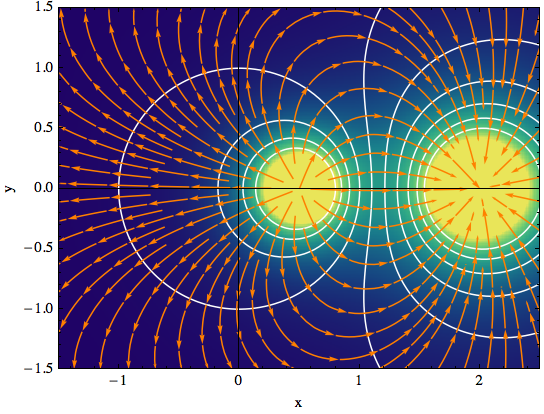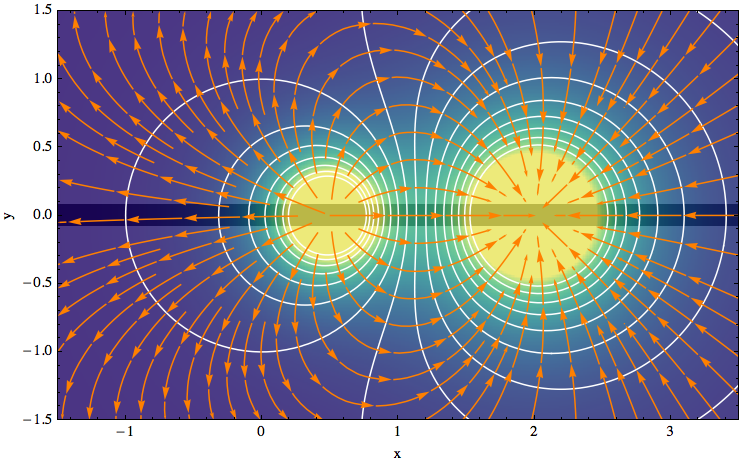# Gradient field plots in Mathematica

This is the HTML version of a Mathematica 8/9 notebook. You can copy and paste the following into a notebook as literal plain text. For the motivation and further discussion of this notebook, see "Mathematica density and contour Plots with rasterized image representation"

### gradientFieldPlot

The function `gradientFieldPlot` takes a scalar potential with two independent variables as its first argument: `φ(x, y)`. The second and third arguments are the name and range of the independent variables, e.g., `{x, xmin, xmax}` and `{y, ymin, ymax}`.

The plot contains three elements:

• a contour plot of the potential `φ`,
• a colored density plot of the gradient field, `∇φ`,
• and a stream plot illustrating the field lines of `∇φ` (they are everywhere perpendicular to the contour lines of the potential).
```gradientFieldPlot[f_, rx_, ry_, opts : OptionsPattern[]] :=
Module[
{
img,
cont,
densityOptions,
contourOptions,
frameOptions,
gradField,
field,
fieldL,
plotRangeRule,
rangeCoords
},
densityOptions = Join[
FilterRules[{opts},
FilterRules[Options[DensityPlot],
Except[{Prolog, Epilog, FrameTicks, PlotLabel, ImagePadding, GridLines, Mesh, AspectRatio, PlotRangePadding, Frame,
Axes}]]],
{PlotRangePadding -> None, Frame -> None, Axes -> None,
AspectRatio -> Automatic}
];
contourOptions = Join[
FilterRules[{opts},
FilterRules[Options[ContourPlot],
Except[{Prolog, Epilog, FrameTicks, PlotLabel, Background, ContourShading, PlotRangePadding, Frame,
Axes, ExclusionsStyle}]]],
{PlotRangePadding -> None, Frame -> None, Axes -> None,
ContourShading -> False}
];
gradField = ComplexExpand[{D[f, rx[]], D[f, ry[]]}];
fieldL =
DensityPlot[Norm[gradField], rx, ry,
Evaluate@Apply[Sequence, densityOptions]];
field=First@Cases[{fieldL},Graphics[__],\[Infinity]];
img = Rasterize[field, "Image"];
plotRangeRule = FilterRules[Quiet@AbsoluteOptions[field], PlotRange];
cont = If[
MemberQ[{0, None}, (Contours /. FilterRules[{opts}, Contours])],
{},
ContourPlot[f, rx, ry,
Evaluate@Apply[Sequence, contourOptions]]
];
frameOptions = Join[
FilterRules[{opts},
FilterRules[Options[Graphics],
Except[{PlotRangeClipping, PlotRange}]]],
{plotRangeRule, Frame -> True, PlotRangeClipping -> True}
];
rangeCoords = Transpose[PlotRange /. plotRangeRule];
If[Head[fieldL]===Legended,Legended[#,fieldL[]],#]&@
Apply[Show[
Graphics[
{
Inset[
Show[SetAlphaChannel[img,
"ShadingOpacity" /. {opts} /. {"ShadingOpacity" -> 1}],
AspectRatio -> Full], rangeCoords[], {0, 0},
rangeCoords[] - rangeCoords[]]
}],
cont,
StreamPlot[gradField, rx, ry,
Evaluate@FilterRules[{opts}, StreamStyle],
Evaluate@FilterRules[{opts}, StreamColorFunction],
Evaluate@FilterRules[{opts}, StreamColorFunctionScaling],
Evaluate@FilterRules[{opts}, StreamPoints],
Evaluate@FilterRules[{opts}, StreamScale]],
##
] &, frameOptions]
]
```

Here is an example of how to use the function. The contour lines of the potential are shown in white, and the streamlines of the gradient field are orange.

```gradientFieldPlot[
(y^2 + (x - 2)^2)^(-1/2) - (y^2 + (x - 1/2)^2)^(-1/2)/2,
{x, -1.5, 2.5}, {y, -1.5, 1.5},
PlotPoints -> 50, ColorFunction -> "BlueGreenYellow", Contours -> 10,
ContourStyle -> White, Frame -> True, FrameLabel -> {"x", "y"},
ClippingStyle -> Automatic, Axes -> True, StreamStyle -> Orange]
```But perhaps you would like to emphasize the horizontal axis by making it really thick. Now you can achieve this without obscuring the plot:

```gradientFieldPlot[(y^2 + (x - 2)^2)^(-1/
2) - (y^2 + (x - 1/2)^2)^(-1/2)/2, {x, -1.5, 3.5}, {y, -1.5, 1.5},
PlotPoints -> 50, ColorFunction -> "BlueGreenYellow", Contours -> 16,
ContourStyle -> White, Frame -> True, FrameLabel -> {"x", "y"},
ClippingStyle -> Automatic, StreamStyle -> Orange, ImageSize -> 500,
GridLinesStyle -> Directive[Thick, Black], "ShadingOpacity" -> .8,
Axes -> {True, False}, AxesStyle -> Directive[Thickness[.03], Black],
Method -> {"AxesInFront" -> False}]
```noeckel@uoregon.edu
Last modified: Tue Aug 19 11:45:21 PDT 2014